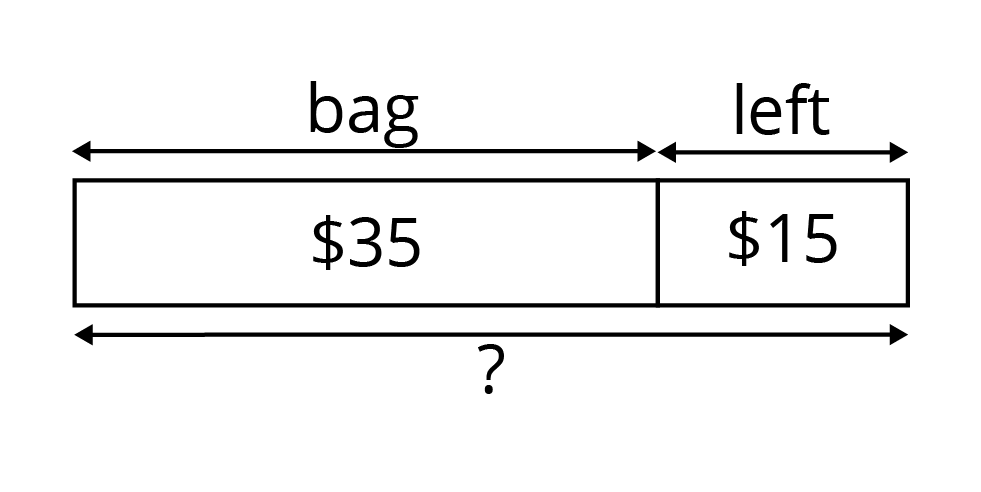# Maths model drawing: Comparison ModelsModel drawing is a highly effective technique for solving word problems, and the comparison model is one of its fundamental components. This model is particularly useful when you need to compare and contrast quantities, helping you break down complex problems into manageable visual representations.

In this comprehensive guide, we'll outline step-by-step instructions on creating comparison models and offer practical examples to illustrate how you can use this model to solve questions.

## Understanding the comparison model

At its core, the comparison model allows you to compare two or more quantities and visualise the relationships between them. It's a versatile tool that can be applied to various scenarios, from counting objects to dealing with financial transactions.

To master the comparison model, follow these key steps:

### Step 1: Identify the key elements

Before you start drawing, carefully read the problem and identify the critical components:

• Quantities: Determine the quantities you need to compare. These could be the number of items, amounts of money, or any other measurable units.
• Persons or groups: Identify the individuals, groups, or entities involved in the comparison. Label them appropriately.
• Relationships: Understand the relationships between the quantities. This includes phrases like "more than," "less than," "equal to," or any other comparison indicators.

Begin by drawing a rectangular box to represent the total quantity. This box is your starting point and serves as a visual anchor for the problem.

### Step 3: Create rectangles for each quantity

For each person or group involved, draw rectangles to represent their quantities. Make sure to label these rectangles with the relevant names.

### Step 4: Indicate the comparison

Pay close attention to the comparison indicators in the problem statement. If someone has more or less of something, use arrows to illustrate this difference. These arrows should extend from one rectangle to another, indicating the direction of the comparison.

### Step 5: Place the question mark

To solve the problem, you need to find an unknown quantity, often represented by a question mark (?). Position the question mark near the appropriate rectangle, indicating that this is the value you need to calculate.

## Examples

Let's dive into some practical examples to demonstrate how the comparison model works in real-world scenarios:

#### Question 1

A bag cost $35$. After buying the bag, Melissa had $15$ left. How much money did she have at first?

Let's solve this using the comparison model method

Step 1: Let's identify the key elements. We can see that the main element of this question is money. We say this because we have been told how much a bag costs ($35$). And we have been told how much money Melissa had left after buying the bag ($15$). We also know that we are expected to find the amount of money she had before she bought the bag.

Step 2: For this example, we have to find the total. Since we don't know the total, we draw an arrow and write '?" in place of the total.

Step 3: Now, we create rectangles for each quantity that we know.

The first quantity is $35$ for a bag, so we create a rectangle on top of the total arrow and write '$35' inside it. We also draw an arrow on top of the rectangle and mention "bag". Next, we know that Melissa was left with $15$ after paying for the bag. So, we draw another rectangle after the bag rectangle and mention '$15' inside it and indicate "left" on top.

By now, our model should look like this:Now, let's solve this model.

We know that

$35+$15= $50 So, we can say that Melissa had$50 before she bought the bag.

Now, let's try a question that's slightly more complicated.

#### Question 2

Phoebe had 812 more stamps than Rachel at first. After Phoebe bought 804 more stamps, she had 5 times as many stamps as Rachel. How many stamps did Rachel have?

Solution:

Following the above steps, we can create a comparison model like this:So, after drawing the model, we can solve the question in the following way:

Difference between Phoebe and Rachel’s stamps in the end

= 812 + 804

= 1616

This means that the value of 4 units = 1616

So, 1 unit would be 1616 $\div$ 4, which is 404.

Since Rachel had 1 unit worth of stamps, we can say that Rachel had 404 stamps.

We hope that these explanations and examples have assisted you in understanding how to solve questions using the comparison Maths model drawing technique. To explore the techniques employed in Maths model drawing, click here.

Exam PreparationPSLEResourcesGCE O-LevelResourcesGCE A-LevelResourcesLatest ArticlesExam PapersPrimarySecondaryWorksheetsPrimaryAEISResourcesLatest ArticlesIGCSEResourcesLatest Articles
Book a free product demo
Suitable for primary & secondaryOur Education Consultants will get in touch with you to offer your child a complimentary Strength Analysis.
Book a free product demo
Suitable for primary & secondary